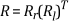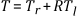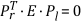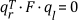# Stereo Calibration In-Depth

NI Vision 2019 for LabVIEW Help

Edition Date: March 2019

Part Number: 370281AG-01

»View Product InfoVision Developoment Module 2019 HelpVision Development Module 2020 Help

You must perform camera model calibration for each camera in the system before performing stereo calibration. Creating a camera model involves acquiring multiple images, usually of a calibration grid, in multiple planes.

For each plane, a camera model provides a set which consists of rotation and translation matrices. Corresponding sets, computed for the left and right camera based on the same plane, provide the information required to compute the spatial relationship between the two cameras. Stereo calibration returns a single rotation and translation matrix (R, T) that relates relative real-world coordinates for the left and right cameras.

The following figure illustrates a calibrated stereo vision system:where
Ol is the origin of the coordinate systems centered at the left camera principal point.

Or is the origin of the coordinate systems centered at the right camera principal point.

P is a real-world point being imaged by both cameras, in real-world coordinates.

pl is the projection of P  on the left-camera image plane.

pr is the projection of P  on the right-camera image plane.

A camera model provides enough information to describe the relationship of the camera relative to a point (P) under inspection. Let, (Rl, Tl) and (Rr, Tr) be the rotation and translation matrices for the left and right cameras for the plane in which P lies. The real-world coordinates of the point P relative to the left and right cameras are given by the following equations:

Pl = RlP + Tl

Pr = RrP + Tr

The 3D coordinates of P are given by the following equation:

Pl = RT(Pr - T).

The stereo rotation matrix is given by the following equation:The stereo translation matrix is given by the following equation:After each camera is calibrated, the stereo vision system must be calibrated.

Stereo calibration computes the essential matrix (E) and the fundamental matrix (F). The essential matrix (E) contains the rotation and translation information required to relate the location of a point (Pl) as seen by the left camera to the same point (Pr) as seen by the right camera, in real-world coordinates. Assuming the relationship Pr = R(PlT), the relationship between points relative to the left and right cameras and the essential matrix is given by the following equation:The essential matrix does not contain information about the internal parameters of the cameras; therefore, it cannot be used to correlate pixel coordinates for conjugate points.
You must use the fundamental matrix (F) to relate pixel coordinates for conjugate points. The fundamental matrix (F) includes internal parameters for both cameras. Give a pixel point in the left image (ql), a conjugate pixel point in the right image (qr), and the fundamental matrix (F), you can compute the corresponding epipolar line in the right image using the following equation: